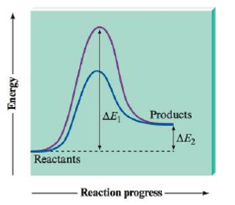# Consider the following energy plots for a chemical reaction when answering the questions below. a. Which plot (purple or blue) is the catalyzed pathway? How do you know? b. What does ∆ E 1 represent? c. What does ∆ E 2 represent? d. Is the reaction endothermic or exothermic?### Chemistry: An Atoms First Approach

2nd Edition
Steven S. Zumdahl + 1 other
Publisher: Cengage Learning
ISBN: 9781305079243

#### Solutions

Chapter
Section### Chemistry: An Atoms First Approach

2nd Edition
Steven S. Zumdahl + 1 other
Publisher: Cengage Learning
ISBN: 9781305079243
Chapter 11, Problem 20Q
Textbook Problem
86 views

## Consider the following energy plots for a chemical reaction when answering the questions below.a. Which plot (purple or blue) is the catalyzed pathway? How do you know?b. What does ∆E1 represent?c. What does ∆E2 represent?d. Is the reaction endothermic or exothermic?

(a)

Interpretation Introduction

Interpretation: Energy plots for a chemical reaction are given. The answers to questions related to the given plots are to be stated.

Concept introduction: The reaction that leads to the release of energy is known as an exothermic reaction, whereas the reaction in which energy is absorbed is known as an endothermic reaction. A catalyst is a substance that increases the rate of a reaction without itself taking part in the reaction. This process is termed as catalysis.

### Explanation of Solution

To determine: The plot corresponding to a catalyzed pathway.

Draw the graph and explain about the plots.

The given plot is,

Figure 1

A catalyst is used to speed up the reaction process...

(b)

Interpretation Introduction

Interpretation: Energy plots for a chemical reaction are given. The answers to questions related to the given plots are to be stated.

Concept introduction: The reaction that leads to the release of energy is known as an exothermic reaction, whereas the reaction in which energy is absorbed is known as an endothermic reaction. A catalyst is a substance that increases the rate of a reaction without itself taking part in the reaction. This process is termed as catalysis.

(c)

Interpretation Introduction

Interpretation: Energy plots for a chemical reaction are given. The answers to questions related to the given plots are to be stated.

Concept introduction: The reaction that leads to the release of energy is known as an exothermic reaction, whereas the reaction in which energy is absorbed is known as an endothermic reaction. A catalyst is a substance that increases the rate of a reaction without itself taking part in the reaction. This process is termed as catalysis.

(d)

Interpretation Introduction

Interpretation: Energy plots for a chemical reaction are given. The answers to questions related to the given plots are to be stated.

Concept introduction: The reaction that leads to the release of energy is known as an exothermic reaction, whereas the reaction in which energy is absorbed is known as an endothermic reaction. A catalyst is a substance that increases the rate of a reaction without itself taking part in the reaction. This process is termed as catalysis.

### Still sussing out bartleby?

Check out a sample textbook solution.

See a sample solution

#### The Solution to Your Study Problems

Bartleby provides explanations to thousands of textbook problems written by our experts, many with advanced degrees!

Get Started

Find more solutions based on key concepts
How do eating disorders affect health?

Understanding Nutrition (MindTap Course List)

Cod is one of the richest sources of the beneficial fatty acids, EPA and DHA. T F

Nutrition: Concepts and Controversies - Standalone book (MindTap Course List)

Provide two reasons why meiosis leads to genetic variation in diploid organisms.

Human Heredity: Principles and Issues (MindTap Course List)

Match the term listed in Column A with its definition from Column B

Nutrition Through the Life Cycle (MindTap Course List)

Two handheld radio transceivers with dipole antennas are separated by a large, fixed distance. If the transmitt...

Physics for Scientists and Engineers, Technology Update (No access codes included)